# Golden ratio

(diff) ← Older revision | Latest revision (diff) | Newer revision → (diff)

golden section, sectio aurea, sectio divina, divina proportio, golden mean, harmonic division, division in extreme and mean ratio

The division of a line segmentinto two parts the greater of which,, is the mean proportional between the whole segmentand the smaller part, i.e.(*)

To find, one has to solve a quadratic equation,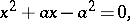the positive solution of which is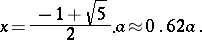Condition (*) may also be written asor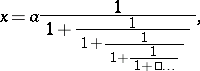i.e. representingas a continued fraction, the convergents of which arewhere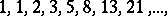are the Fibonacci numbers.Figure: g044570a

The golden ratio of a segmentmay be found by a geometric construction (see Fig.). Draw the perpendicular to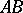at; on the perpendicular, mark off the segment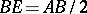; then joinand, mark offand finally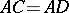. ThenThe golden ratio was already known in Antiquity. Its first appearance in the extant classical literature is in Euclid's Elements, Book II, 11 (3th century B.C.).

Principles based on the golden ratio or similar proportional ratios provided the basis for the compositional design of many works of art (mainly architectural works of Antiquity and the Renaissance).